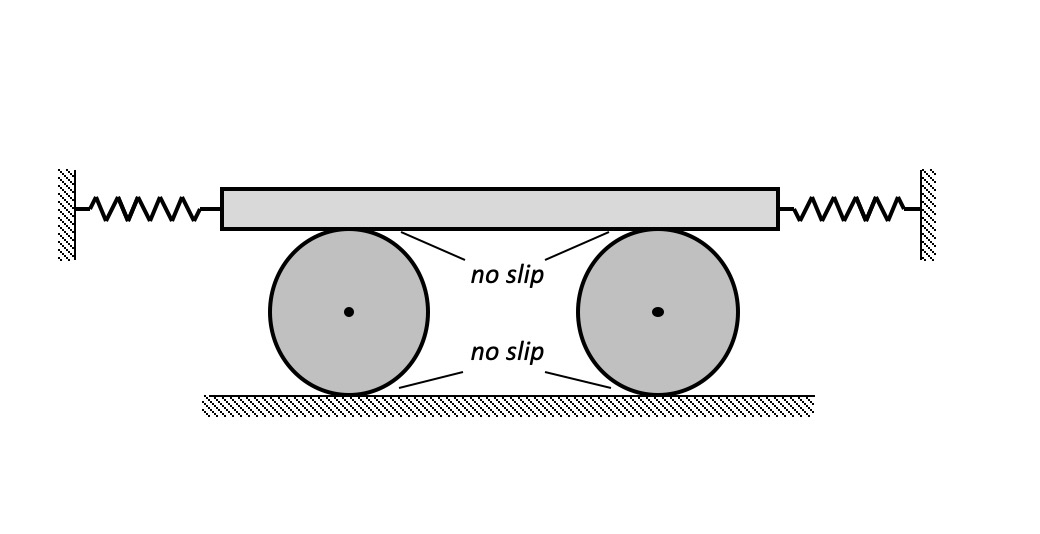# Drawing FBDsOne of the major challenges in the study of vibrations is drawing free body diagrams (FBDs) that are valid for all subsequent motion of the system. Spring forces must be drawn in directions that correctly represent that compressedsprings push and stretched springs pull (the directions drawn in your FBD will depend on your choice of coordinates). The direction of friction forces due to rolling without slipping are not known in advance (since these directions switch over one period of oscillations) – it is important to always abide by Newton’s 3rd law (equal and opposite force pairs) and let math determine the correct directions at any instant.

Animation

The animation below demonstrates how the direction of friction forces due to rolling switch during a given period of oscillations. This animation also shows how the direction of spring forces change when the spring moves from tension to compression and back. Determination of the actual directions of the friction forces is more complicated since these forces depend on accelerations, rather than displacements alone.IMPORTANT NOTE:
You do NOT need to know the direction of non-slip friction forces at the time of drawing FBD's. Simply treat these as regular reaction forces, and as long as you abide by Newton's Third Law (equal and opposite forces), the mathematics will take care of the signs of these forces of friction.